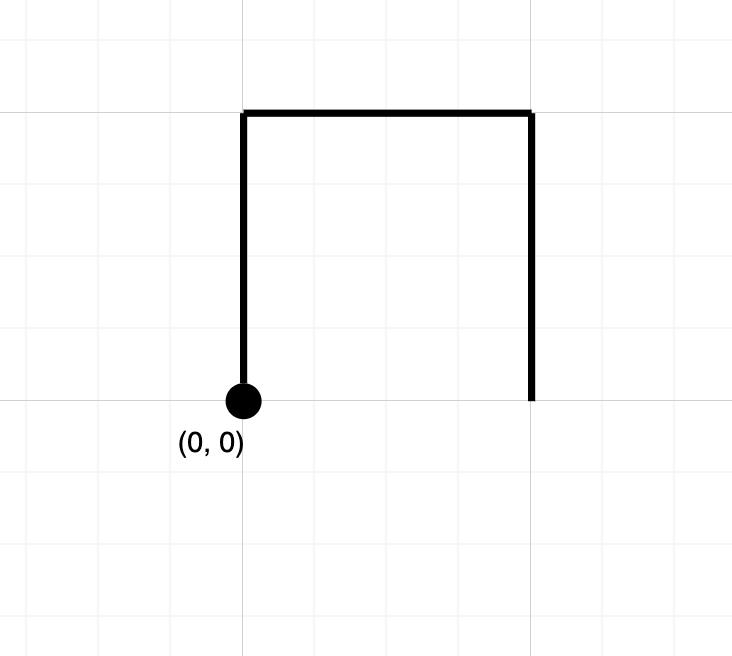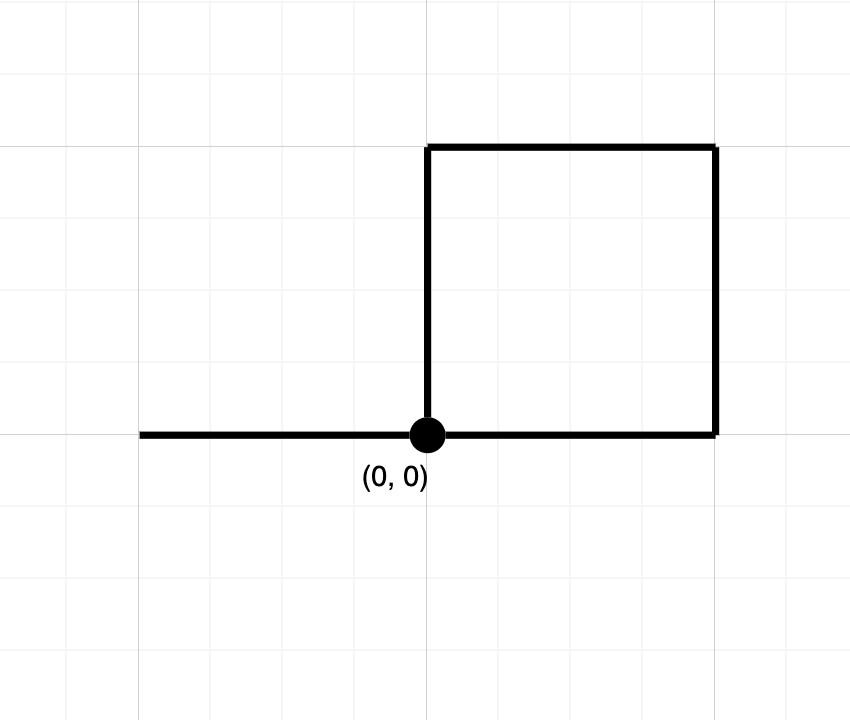Formatted question description: https://leetcode.ca/all/1496.html

# 1496. Path Crossing (Easy)

Given a string path, where path[i] = 'N', 'S', 'E' or 'W', each representing moving one unit north, south, east, or west, respectively. You start at the origin (0, 0) on a 2D plane and walk on the path specified by path.

Return True if the path crosses itself at any point, that is, if at any time you are on a location you've previously visited. Return False otherwise.

Example 1:Input: path = "NES"
Output: false
Explanation: Notice that the path doesn't cross any point more than once.


Example 2:Input: path = "NESWW"
Output: true
Explanation: Notice that the path visits the origin twice.

Constraints:

• 1 <= path.length <= 10^4
• path will only consist of characters in {'N', 'S', 'E', 'W}

Related Topics:
String

## Solution 1.

// OJ: https://leetcode.com/problems/path-crossing/

// Time: O(N)
// Space: O(N)
class Solution {
long hash(int x, int y) { return (long)x * 10000 + y; }
public:
bool isPathCrossing(string path) {
unordered_set<long> s;
int x = 0, y = 0;
s.insert(hash(x, y));
for (char c : path) {
if (c == 'N') ++y;
else if (c == 'E') ++x;
else if (c == 'S') --y;
else --x;
long key = hash(x, y);
if (s.count(key)) return true;
s.insert(key);
}
return false;
}
};


Java

class Solution {
public boolean isPathCrossing(String path) {
char[] directionChars = {'N', 'S', 'E', 'W'};
int[][] directions = { {0, 1}, {0, -1}, {1, 0}, {-1, 0} };
Map<Character, Integer> map = new HashMap<Character, Integer>();
for (int i = 0; i < 4; i++)
map.put(directionChars[i], i);
Set<String> set = new HashSet<String>();
int x = 0, y = 0;
int length = path.length();
for (int i = 0; i < length; i++) {
char c = path.charAt(i);
int index = map.get(c);
int[] direction = directions[index];
x += direction;
y += direction;
int[] array = {x, y};